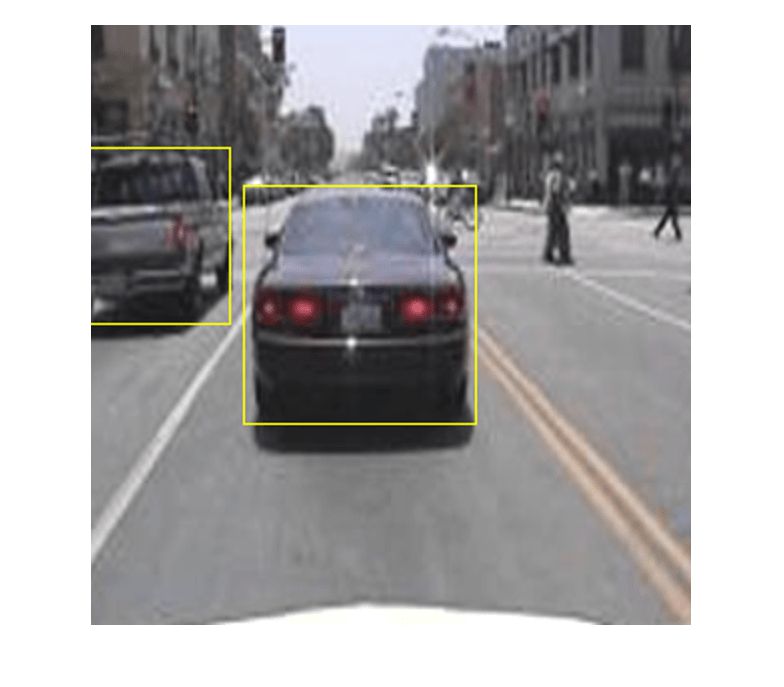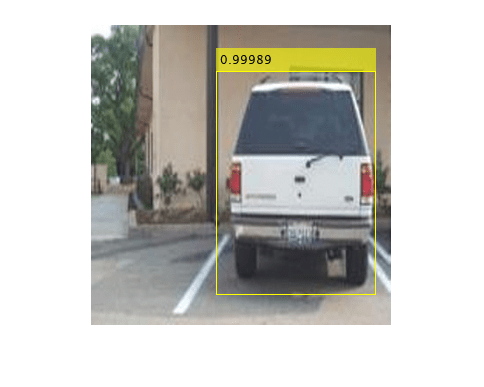# Object Detection Using SSD Deep Learning

This example shows how to train a Single Shot Detector (SSD).

### Overview

Deep learning is a powerful machine learning technique that automatically learns image features required for detection tasks. There are several techniques for object detection using deep learning such as Faster R-CNN, You Only Look Once (YOLO v2), and SSD. This example trains an SSD vehicle detector using the `trainSSDObjectDetector` function. For more information, see Object Detection (Computer Vision Toolbox).

Download a pretrained detector to avoid having to wait for training to complete. If you want to train the detector, set the `doTraining` variable to true.

```doTraining = false; if ~doTraining && ~exist('ssdResNet50VehicleExample_20a.mat','file') disp('Downloading pretrained detector (44 MB)...'); pretrainedURL = 'https://www.mathworks.com/supportfiles/vision/data/ssdResNet50VehicleExample_20a.mat'; websave('ssdResNet50VehicleExample_20a.mat',pretrainedURL); end```
```Downloading pretrained detector (44 MB)... ```

This example uses a small vehicle data set that contains 295 images. Many of these images come from the Caltech Cars 1999 and 2001 data sets, created by Pietro Perona and used with permission. Each image contains one or two labeled instances of a vehicle. A small data set is useful for exploring the SSD training procedure, but in practice, more labeled images are needed to train a robust detector.

```unzip vehicleDatasetImages.zip data = load('vehicleDatasetGroundTruth.mat'); vehicleDataset = data.vehicleDataset;```

The training data is stored in a table. The first column contains the path to the image files. The remaining columns contain the ROI labels for vehicles. Display the first few rows of the data.

`vehicleDataset(1:4,:)`
```ans=4×2 table imageFilename vehicle _________________________________ _________________ {'vehicleImages/image_00001.jpg'} {[220 136 35 28]} {'vehicleImages/image_00002.jpg'} {[175 126 61 45]} {'vehicleImages/image_00003.jpg'} {[108 120 45 33]} {'vehicleImages/image_00004.jpg'} {[124 112 38 36]} ```

Split the data set into a training set for training the detector and a test set for evaluating the detector. Select 60% of the data for training. Use the rest for evaluation.

```rng(0); shuffledIndices = randperm(height(vehicleDataset)); idx = floor(0.6 * length(shuffledIndices) ); trainingData = vehicleDataset(shuffledIndices(1:idx),:); testData = vehicleDataset(shuffledIndices(idx+1:end),:);```

Use `imageDatastore` and `boxLabelDatastore` to load the image and label data during training and evaluation.

```imdsTrain = imageDatastore(trainingData{:,'imageFilename'}); bldsTrain = boxLabelDatastore(trainingData(:,'vehicle')); imdsTest = imageDatastore(testData{:,'imageFilename'}); bldsTest = boxLabelDatastore(testData(:,'vehicle'));```

Combine image and box label datastores.

```trainingData = combine(imdsTrain,bldsTrain); testData = combine(imdsTest, bldsTest);```

Display one of the training images and box labels.

```data = read(trainingData); I = data{1}; bbox = data{2}; annotatedImage = insertShape(I,'Rectangle',bbox); annotatedImage = imresize(annotatedImage,2); figure imshow(annotatedImage)```### Create a SSD Object Detection Network

The SSD object detection network can be thought of as having two sub-networks. A feature extraction network, followed by a detection network.

The feature extraction network is typically a pretrained CNN (see Pretrained Deep Neural Networks for more details). This example uses ResNet-50 for feature extraction. Other pretrained networks such as MobileNet v2 or ResNet-18 can also be used depending on application requirements. The detection sub-network is a small CNN compared to the feature extraction network and is composed of a few convolutional layers and layers specific to SSD.

Use the `ssdLayers` function to automatically modify a pretrained ResNet-50 network into a SSD object detection network. `ssdLayers` requires you to specify several inputs that parameterize the SSD network, including the network input size and the number of classes. When choosing the network input size, consider the size of the training images, and the computational cost incurred by processing data at the selected size. When feasible, choose a network input size that is close to the size of the training image. However, to reduce the computational cost of running this example, the network input size is chosen to be [300 300 3]. During training, `trainSSDObjectDetector` automatically resizes the training images to the network input size.

`inputSize = [300 300 3];`

Define number of object classes to detect.

`numClasses = width(vehicleDataset)-1;`

Create the SSD object detection network.

`lgraph = ssdLayers(inputSize, numClasses, 'resnet50');`

You can visualize the network using `analyzeNetwork` or D`eepNetworkDesigner` from Deep Learning Toolbox™. Note that you can also create a custom SSD network layer-by-layer. For more information, see Create SSD Object Detection Network (Computer Vision Toolbox).

### Data Augmentation

Data augmentation is used to improve network accuracy by randomly transforming the original data during training. By using data augmentation, you can add more variety to the training data without actually having to increase the number of labeled training samples. Use `transform` to augment the training data by

• Randomly flipping the image and associated box labels horizontally.

• Randomly scale the image, associated box labels.

• Jitter image color.

Note that data augmentation is not applied to the test data. Ideally, test data should be representative of the original data and is left unmodified for unbiased evaluation.

`augmentedTrainingData = transform(trainingData,@augmentData);`

Visualize augmented training data by reading the same image multiple times.

```augmentedData = cell(4,1); for k = 1:4 data = read(augmentedTrainingData); augmentedData{k} = insertShape(data{1},'Rectangle',data{2}); reset(augmentedTrainingData); end figure montage(augmentedData,'BorderSize',10)```### Preprocess Training Data

Preprocess the augmented training data to prepare for training.

`preprocessedTrainingData = transform(augmentedTrainingData,@(data)preprocessData(data,inputSize));`

`data = read(preprocessedTrainingData);`

Display the image and bounding boxes.

```I = data{1}; bbox = data{2}; annotatedImage = insertShape(I,'Rectangle',bbox); annotatedImage = imresize(annotatedImage,2); figure imshow(annotatedImage)```### Train SSD Object Detector

Use `trainingOptions` to specify network training options. Set `'CheckpointPath'` to a temporary location. This enables the saving of partially trained detectors during the training process. If training is interrupted, such as by a power outage or system failure, you can resume training from the saved checkpoint.

```options = trainingOptions('sgdm', ... 'MiniBatchSize', 16, .... 'InitialLearnRate',1e-1, ... 'LearnRateSchedule', 'piecewise', ... 'LearnRateDropPeriod', 30, ... 'LearnRateDropFactor', 0.8, ... 'MaxEpochs', 300, ... 'VerboseFrequency', 50, ... 'CheckpointPath', tempdir, ... 'Shuffle','every-epoch');```

Use `trainSSDObjectDetector` (Computer Vision Toolbox) function to train SSD object detector if `doTraining` to true. Otherwise, load a pretrained network.

```if doTraining % Train the SSD detector. [detector, info] = trainSSDObjectDetector(preprocessedTrainingData,lgraph,options); else % Load pretrained detector for the example. pretrained = load('ssdResNet50VehicleExample_20a.mat'); detector = pretrained.detector; end```

This example is verified on an NVIDIA™ Titan X GPU with 12 GB of memory. If your GPU has less memory, you may run out of memory. If this happens, lower the '`MiniBatchSize`' using the `trainingOptions` function. Training this network took approximately 2 hours using this setup. Training time varies depending on the hardware you use.

As a quick test, run the detector on one test image.

```data = read(testData); I = data{1,1}; I = imresize(I,inputSize(1:2)); [bboxes,scores] = detect(detector,I, 'Threshold', 0.4);```

Display the results.

```I = insertObjectAnnotation(I,'rectangle',bboxes,scores); figure imshow(I)```### Evaluate Detector Using Test Set

Evaluate the trained object detector on a large set of images to measure the performance. Computer Vision Toolbox™ provides object detector evaluation functions to measure common metrics such as average precision (`evaluateDetectionPrecision`) and log-average miss rates (`evaluateDetectionMissRate`). For this example, use the average precision metric to evaluate performance. The average precision provides a single number that incorporates the ability of the detector to make correct classifications (`precision`) and the ability of the detector to find all relevant objects (`recall`).

Apply the same preprocessing transform to the test data as for the training data. Note that data augmentation is not applied to the test data. Test data should be representative of the original data and be left unmodified for unbiased evaluation.

`preprocessedTestData = transform(testData,@(data)preprocessData(data,inputSize));`

Run the detector on all the test images.

`detectionResults = detect(detector, preprocessedTestData, 'Threshold', 0.4);`

Evaluate the object detector using average precision metric.

`[ap,recall,precision] = evaluateDetectionPrecision(detectionResults, preprocessedTestData);`

The precision/recall (PR) curve highlights how precise a detector is at varying levels of recall. Ideally, the precision would be 1 at all recall levels. The use of more data can help improve the average precision, but might require more training time Plot the PR curve.

```figure plot(recall,precision) xlabel('Recall') ylabel('Precision') grid on title(sprintf('Average Precision = %.2f',ap))```### Code Generation

Once the detector is trained and evaluated, you can generate code for the `ssdObjectDetector` using GPU Coder™. For more details, see Code Generation for Object Detection by Using Single Shot Multibox Detector (Computer Vision Toolbox) example.

### Supporting Functions

```function B = augmentData(A) % Apply random horizontal flipping, and random X/Y scaling. Boxes that get % scaled outside the bounds are clipped if the overlap is above 0.25. Also, % jitter image color. B = cell(size(A)); I = A{1}; sz = size(I); if numel(sz)==3 && sz(3) == 3 I = jitterColorHSV(I,... 'Contrast',0.2,... 'Hue',0,... 'Saturation',0.1,... 'Brightness',0.2); end % Randomly flip and scale image. tform = randomAffine2d('XReflection',true,'Scale',[1 1.1]); rout = affineOutputView(sz,tform,'BoundsStyle','CenterOutput'); B{1} = imwarp(I,tform,'OutputView',rout); % Sanitize boxes, if needed. A{2} = helperSanitizeBoxes(A{2}, sz); % Apply same transform to boxes. [B{2},indices] = bboxwarp(A{2},tform,rout,'OverlapThreshold',0.25); B{3} = A{3}(indices); % Return original data only when all boxes are removed by warping. if isempty(indices) B = A; end end function data = preprocessData(data,targetSize) % Resize image and bounding boxes to the targetSize. sz = size(data{1},[1 2]); scale = targetSize(1:2)./sz; data{1} = imresize(data{1},targetSize(1:2)); % Sanitize boxes, if needed. data{2} = helperSanitizeBoxes(data{2}, sz); % Resize boxes. data{2} = bboxresize(data{2},scale); end```

### References

 Liu, Wei, Dragomir Anguelov, Dumitru Erhan, Christian Szegedy, Scott Reed, Cheng Yang Fu, and Alexander C. Berg. "SSD: Single shot multibox detector." In 14th European Conference on Computer Vision, ECCV 2016. Springer Verlag, 2016.